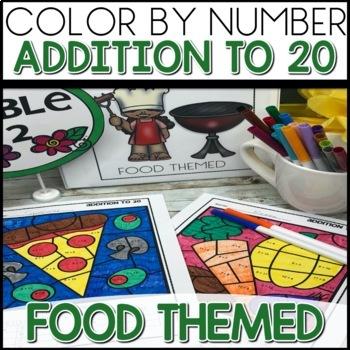# Color by Number Math Worksheets Food Themed BundleK - 2nd
Subjects
Resource Type
Standards
Formats Included
• Zip
Pages
35 pages
\$12.00
List Price:
\$15.00
You Save:
\$3.00
Bundle
\$12.00
List Price:
\$15.00
You Save:
\$3.00
Bundle
Report this resource to TPT

#### Products in this Bundle (5)

##### Also included in
1. If you are looking for a huge bundle of color by number addition and subtraction math coloring pages, this bundle has it all! These fun math worksheets have everything you need to keep your students actively engaged as they practice addition to 12, addition to 20, subtraction within 20, addition an
Price \$94.50Original Price \$135.00Save \$40.50

### Description

Your students will be actively engaged as they practice addition to 12, addition to 20, subtraction within 20, mixed review to 20, and missing addends with the resource! There's something for all of your students, depending on where they are in the learning process. This is a Food Themed resource!

Save 30% on MEGA Bundle!

What's included:

Other Food Themed Color-by-Code resources:

COLOR BY NUMBER ACTIVITIES

These are a fun way for your students to practice math strategies, while working on fine motor skills. If your students LOVE color by code worksheets, I have a ton of different themes. Click here to see them all! These are perfect for classroom activities, morning work, center activities, and homework.

WHO LIKES A DEAL?

• Follow me and be notified when new products are uploaded. New products are always 50% off for the first 24 hours they are posted (except for resources that are under \$1.50). It pays to follow me!

QUESTIONS OR CONCERNS

I sure appreciate you!

Shanon Juneau ❤️

Total Pages
35 pages
Included
Teaching Duration
Lifelong tool
Report this resource to TPT
Reported resources will be reviewed by our team. Report this resource to let us know if this resource violates TPT’s content guidelines.

### Standards

to see state-specific standards (only available in the US).
Apply properties of operations as strategies to add and subtract. If 8 + 3 = 11 is known, then 3 + 8 = 11 is also known. (Commutative property of addition.) To add 2 + 6 + 4, the second two numbers can be added to make a ten, so 2 + 6 + 4 = 2 + 10 = 12. (Associative property of addition.)
Understand subtraction as an unknown-addend problem. For example, subtract 10 – 8 by finding the number that makes 10 when added to 8.
Add and subtract within 20, demonstrating fluency for addition and subtraction within 10. Use strategies such as counting on; making ten (e.g., 8 + 6 = 8 + 2 + 4 = 10 + 4 = 14); decomposing a number leading to a ten (e.g., 13 - 4 = 13 - 3 - 1 = 10 - 1 = 9); using the relationship between addition and subtraction (e.g., knowing that 8 + 4 = 12, one knows 12 - 8 = 4); and creating equivalent but easier or known sums (e.g., adding 6 + 7 by creating the known equivalent 6 + 6 + 1 = 12 + 1 = 13).
Understand the meaning of the equal sign, and determine if equations involving addition and subtraction are true or false. For example, which of the following equations are true and which are false? 6 = 6, 7 = 8 - 1, 5 + 2 = 2 + 5, 4 + 1 = 5 + 2.
Determine the unknown whole number in an addition or subtraction equation relating three whole numbers. For example, determine the unknown number that makes the equation true in each of the equations 8 + ? = 11, 5 = ▯ - 3, 6 + 6 = ▯.